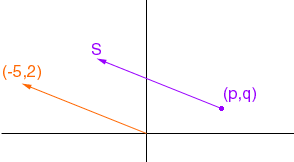My name is Jennifer. I am a student and can't figure out a certain problem that my teacher gave me. I am in the 10th grade. The question is: Give the image of (p,q) when translated by the vector (-5,2). Please help me with this. Thank you. -Jennifer Hi Jennifer, Draw the vector as an arrow from the orogin and think of it as an action that moves every point in the direction of the arrow and a distance of the length of the arrow.Thus the point (p,q) is moved five units to the left and two units upward, to the point S in the diagram. Hence the x-coordinate of S is 5 less than the x-coordinate of (p,q) and the y-coordinate of S is 2 units more than the y-coordinate of (p,q). That is S has coordinates (p - 5, q + 2). Cheers, Penny Go to Math Central